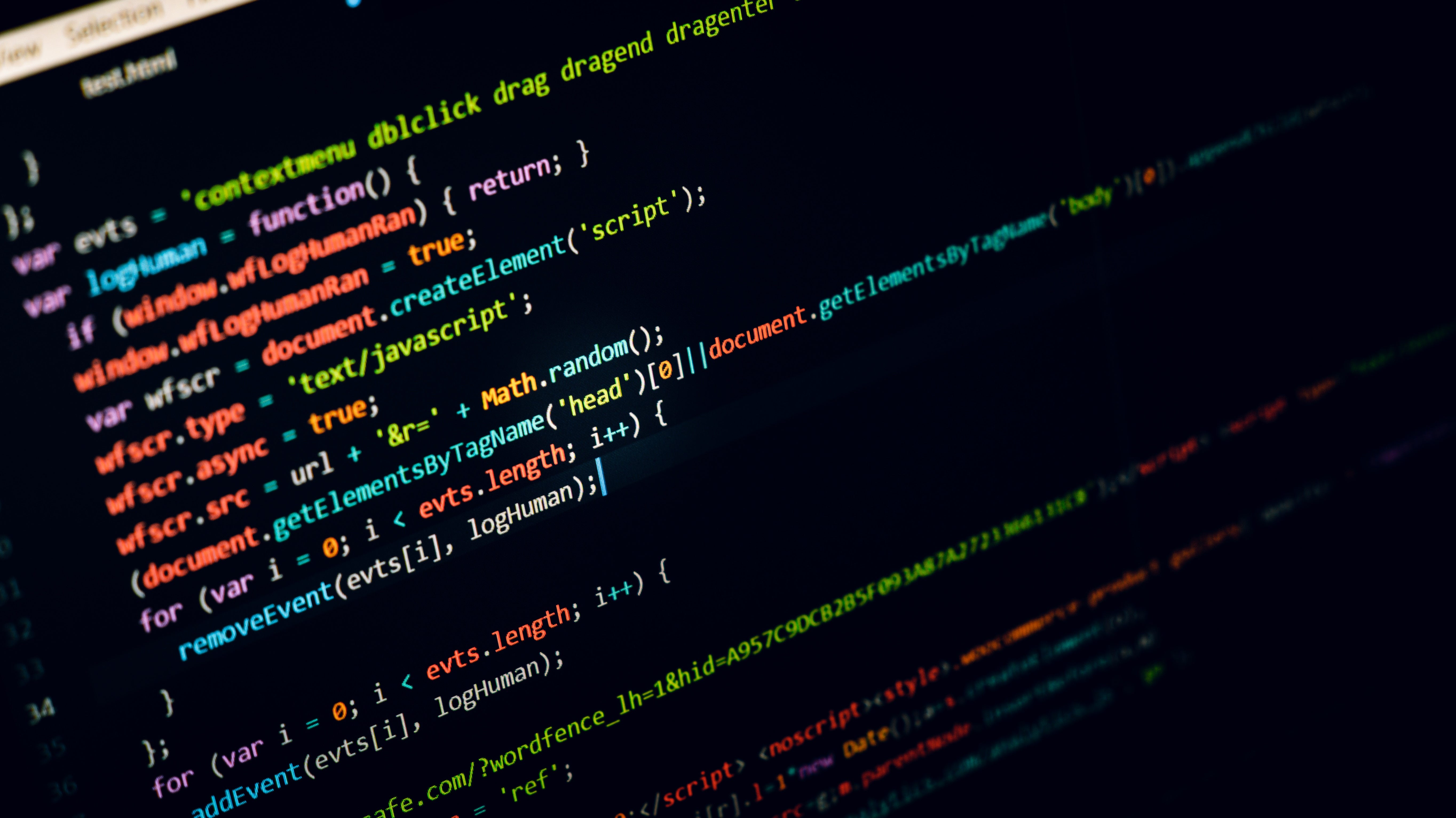Photo by Dlanor S on Unsplash

# How to get upvotes for a kernel on Kaggle?

## An analysis of Kaggle’s public data

I have recently joined Kaggle and started to create public kernels. My kernels have a lot of views, but no upvotes. Fortunately, there is Meta Kaggle dataset, which contains various data on competitions, users, submissions, and kernels. We can use this dataset to find out:

• Statistics for the number of kernels, which have votes;

In this article, I will just share my findings, the full code for the analysis you can find on GitHub or in Kaggle kernel.

## Explore statistics for the number of kernels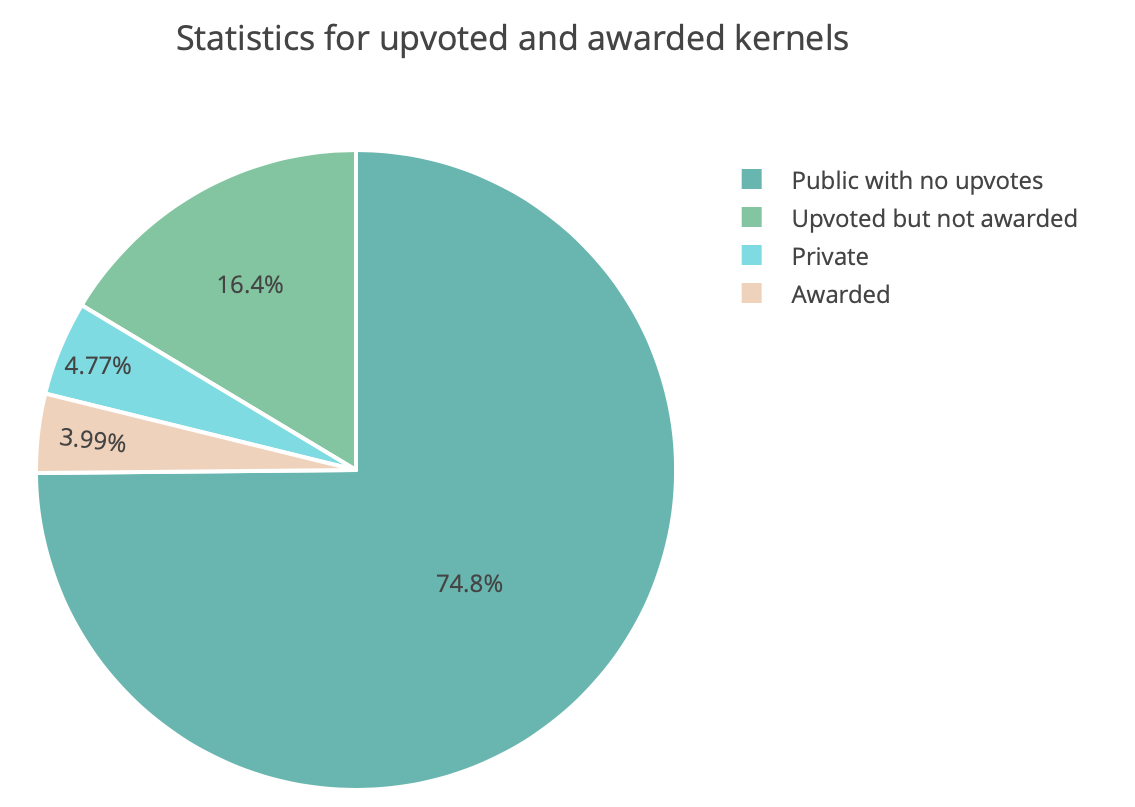Plot 1. Kernel statistics pie chart

If we just count the total number of kernels and compare it to the number of ones which have upvotes, we can see that writing a popular kernel is not an easy task.

There are more than 220 thousand kernels on Kaggle in total, only 20% of them were upvoted by Kaggle users, and only 4% are awarded, which means has more than 5 upvotes (see Plot 1).

## How does the number of views and number of comments affect the number of votes?

My idea is that the more people view or discuss the kernel, the more votes it gets. To prove this assumption let’s first look at the correlation between the number of votes and the number of views and comments: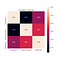Plot 2. Correlation between the number of votes and the number of views and comments

We really see that these numbers are highly correlated. We can also plot the number of votes versus the number of views and comments and add to the plot the linear line, which shows the dependency between them: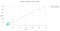Plot 3. The number of votes per number of views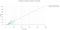Plot 4. The number of votes per number of comments

Looking at the plots it really seems that my idea was right. To gain upvotes from the users, the kernel needs to be shared with others, seen and discussed.

## How does the status of the author affect the number of votes per kernel?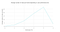Plot 5. The average number of votes depending on user performance tier

Kaggle has its own progression system, there are performance tiers depending on the proficiency and the contribution of the users.

Indeed, we can see on the plot 5 that kernels created by more proficient authors gain more votes on average.

## How does dataset related to kernel affects the number of votes?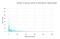Plot 6. The average number of kernel votes per number of dataset downloads

Kernels on Kaggle use as data sources datasets released on Kaggle. Datasets on Kaggle also gain votes from users. Let’s try to find out how the popularity of a dataset affects the number of votes for the related kernels?

I tried to plot the average number of kernel votes depending on the number of votes and downloads for the dataset,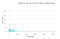Plot 7. The average number of kernel votes per number of votes for a related dataset

which was used as the data source (see plot 6 and plot 7).

It looks like there is no dependency between the number of votes for s kernel and the number of votes or downloads for the dataset used as a data source. I suppose that we can create a really helpful and popular kernel for an unpopular dataset and vice versa.

## How does kernel language affect the number of votes?

Kernels on Kaggle can have different language types, for example, Python scripts, Python notebooks, R scripts etc. The plot below shows the average number of votes for each language type: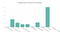Plot 8. The average number of votes per kernel language

It looks like descriptive kernels which use markdown are more appreciated with Kaggle users and gain more votes on average.

## How do kernel tags affect the number of votes?

Authors can add tags to their kernels. We can plot the average number of kernel votes for each of top-20 most popular tags on Kaggle: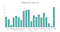Plot 9. Average number of kernel votes per top-20 most popular tags

It is also interesting to find out which tags have the greatest average number of votes: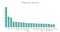Plot 10. Tags with the highest average number of kernel votes

According to the plots, the most kernels tagged with the most popular tags on Kaggle do not score the greatest number of votes.

# Conclusion

In conclusion, I would like to summarize all the findings and recommendations from this analysis:

1. It is hard to create a really helpful kernel, which will be appreciated and upvoted by Kagglers: only 20% of kernels have upvotes and only 4% of kernels have awards (have more than 5 upvotes).

A business analyst turned data scientist passionate about solving business problems with data. Connect me: https://www.linkedin.com/in/aleksandra-deis-0912/

## More from Alexandra Deis

A business analyst turned data scientist passionate about solving business problems with data. Connect me: https://www.linkedin.com/in/aleksandra-deis-0912/